# High Low Method vs. Regression Analysis

Methods used to estimate the amounts of fixed and variable costs

## High Low Method vs. Regression Analysis

The high low method and regression analysis are the two main cost estimation methods used to estimate the amounts of fixed and variable costs. Usually, managers must break mixed costs into their fixed and variable components, to predict and plan for the future.

Learn more in CFI’s Math for Corporate Finance Course.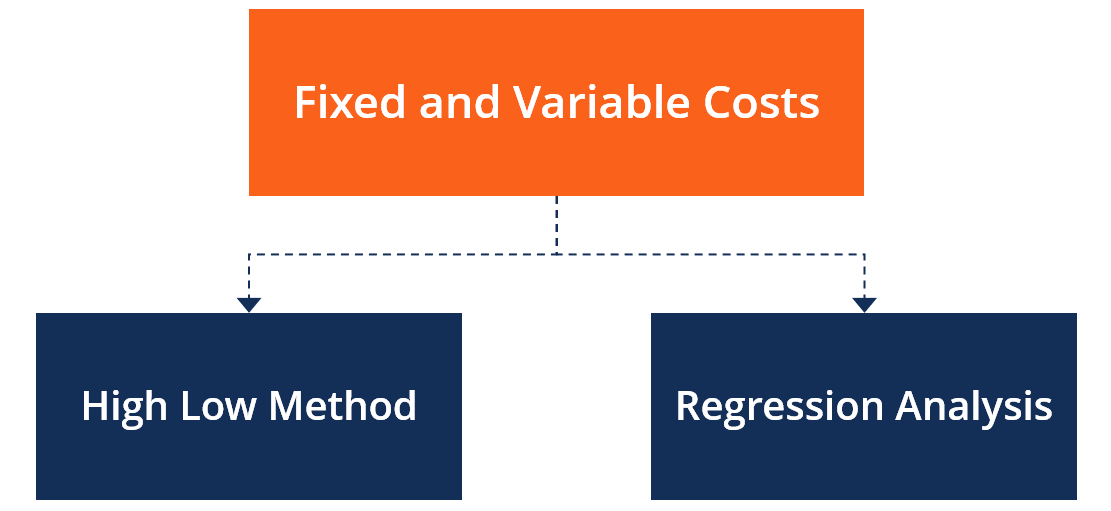The high low method uses a small amount of data to separate fixed and variable costs. It takes the highest and lowest activity levels and compares their total costs. On the other hand, regression analysis shows the relationship between two or more variables. It is used to observe changes in the dependent variable relative to changes in the independent variable.

### High Low Method

The high low method determines the fixed and variable components of a cost. It can be applied in discerning the fixed and variable elements of the cost of a product, machine, store, geographic sales region, product line, etc.

For example, in the production cost of a product, fixed costs may comprise employee’s wages and rental expenses, whereas variable costs include costs incurred in purchasing raw materials.

#### Formula

The high low method splits the variable and fixed components of mixed costs. The formulas for each component are as follows: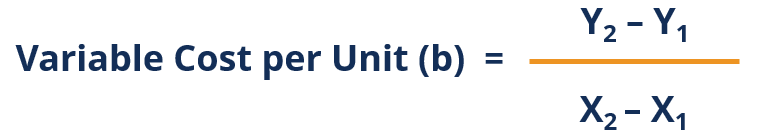Where:

Y2 is the cost at the highest activity level

Y1 is the cost at the lowest activity level

X2 is the number of units at the highest activity level

X1 is the number of units at the lowest activity level

Once the variable cost has been calculated, the fixed cost can be derived by subtracting the total variable cost from the total cost. This is represented by the following formula:

or

##### Fixed Cost = Y1 – bX1

Where:

b is the variable cost

#### Example

Company ABC is a manufacturer of pharmaceuticals. The company wants to estimate the amount of overhead costs that it will incur in April, given that the company plans to make 8,000 units in that month. Following are the figures from January to March: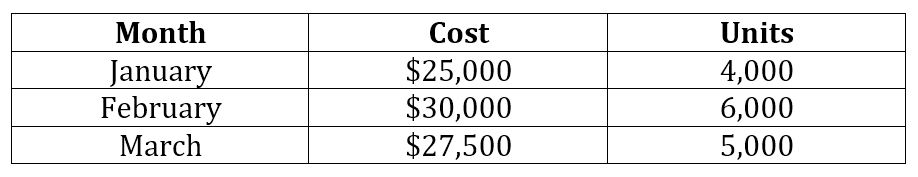Using the variable cost formula above, where:

Y2 = \$30,000

Y1 = \$25,000

X2 = 6,000

X1 = 4,000

Therefore: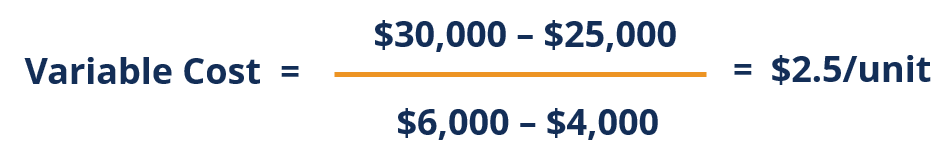or

##### Fixed Cost = \$25,000 – (2.5 x \$4,000) = \$15,000

Projected variable cost for the month of April is calculated as follows:

= 2.5 x \$8,000

= \$20,000

Total cost = Fixed cost + Variable cost

= \$15,000 + \$20,000

= \$35,000

Learn more in CFI’s Math for Corporate Finance Course.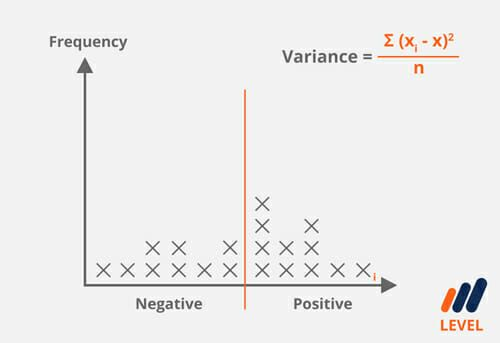### Advantages of High Low Method

Using the high low method offers the following benefits:

#### Easy to use

The high-low method only requires the cost and unit information at the highest and lowest activity level to get the required information. Managers can implement this technique with ease since it does not require any special tools.

#### High accuracy with stable costs

The high low method can be relatively accurate if the highest and lowest activity levels are representative of the overall cost behavior of the company. However, if the two extreme activity levels are systematically different, then the high low method will produce inaccurate results.

### Disadvantages of High Low Method

The high low method comes with the following disadvantages:

#### May be Unreliable

The method does not represent of all the data provided since it relies on just two extreme activity levels. Those activity levels may not be representative of the costs incurred, due to outlier costs that are higher or lower than what the organization incurs in the other activity levels.

#### Does not account for inflation

The high low method excludes the effects of inflation when estimating costs.

### Regression Analysis

In contrast to the High Low Method, Regression analysis refers to the technique for estimating the relationship between variables. It helps people understand how the value of a dependent variable changes when one independent variable is variable while another is held constant. Regression analysis is used in forecasting future data. The two main types of regression analysis are linear regression and multiple regression.

#### Linear regression

Linear regression is a method that studies the relationship between continuous variables. The variables are plotted on a straight line. The linear regression can be calculated using the following formula:

##### Y = a + bX + ⋴

Where:

Y is the dependent variable

X is the independent variable

b is the slope of the regression line

a is the intercept of the regression line

⋴ is the regression residual

From the formula above, we can deduce that the value of Y is dependent on the value of X, while the value of b shows the changes in Y every time the value of X changes. Meaning, if b equals 0.5, it means that every time X increases or increases by a specific value, the value of Y increases or decreases by that value. On the other hand, when X=0, the value of “a” equals the value of Y.

#### Assumptions of Linear Regression

• The relationship between dependent variable Y and independent variable X is linear in the slope and intercept ‘a’ and ‘b,’ respectively.
• Independent variable X is not random.
• The value of the error term ⋴ is 0 and is constant for all observations.

### Multiple Regression

Multiple regression is a statistical technique that predicts the value of one variable using the value of two or more independent variables. Once each of the independent variables has been determined, they can be used to predict the amount of effect that the independent variables have on the dependent variable. The effect is represented on a straight line to approximate each of the data points.

Learn more in CFI’s Math for Corporate Finance Course.#### Formula

The formula for multiple regression is as follows:

##### Y1= B0 + B1x1 + b2x2+ ………..bnxn + ⋴

Where:

Y1 is the predicted value of the dependent variable

B0 is the intercept

B1, b2 … bn are the regression coefficient

x1, x2, …… xn are the independent variables

⋴ is the regression residual

#### Assumptions of Multiple Regression

• There is a linear relationship between dependent and independent variables.
• Y1 observations are selected independently and randomly from the population.
• Independent variables are not highly correlated with each other.
• The regression residual shows a mean of 0 and variance of 0.

Thank you for reading CFI’s comparison of the High Low Method and Regression Analysis. CFI offers the Financial Modeling & Valuation Analyst (FMVA)™ certification program for those looking to take their careers to the next level. To keep learning and advancing your career, the following resources will be helpful:

• Cost Structure
• Homogeneous Cost Pool
• Projecting Income Statement Line Items
• Variable Costing

### Financial Analyst Training

Get world-class financial training with CFI’s online certified financial analyst training program!

Gain the confidence you need to move up the ladder in a high powered corporate finance career path.

Learn financial modeling and valuation in Excel the easy way, with step-by-step training.